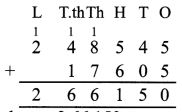# DAV Class 4 Maths Chapter 2 Brain Teasers Solutions

The DAV Class 4 Maths Book Solutions and DAV Class 4 Maths Chapter 2 Brain Teasers Solutions of Addition and Subtraction offer comprehensive answers to textbook questions.

## DAV Class 4 Maths Ch 2 Brain Teasers Solutions

Question 1.
(a) When we add 100 to 9,136, the digit at ___________ place increases by 1.
(i) ones
(ii) hundreds
(iii) tens
(iv) thousands
(ii) hundreds

(b) The greatest 2-digit number is less than the smallest 3-digit number by ___________
(i) 10
(ii) 9
(iii) 1
(iv) 0
(iii) 1

(c) 7,000 – 5,000 – 2,000 ___________.
(i) 0
(ii) 3,000
(iii) 2,000
(iv) 1,000
(i) 0

(d) 400 more than the successor of 399 is equal to ___________.
(i) 401
(ii) 499
(iii) 400
(iv) 800
(iv) 800

(e) 10 + 101 + 1,001 + 10,001 is equal to
(i) 10,013
(ii) 11,113
(iii) 40,111
(iv) 11,001
(ii) 11,113Question 2.
Solve:
(a) 85,781 + 78,989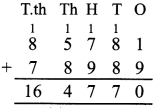(b) 48,125 + 9,999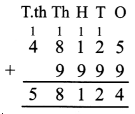(c) 7,56,480 – 51,345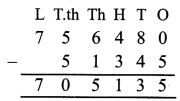(d) 80,000 – 79,899
Arrange in column then solve.Question 3.
Write down the largest number and the smallest number of four digits in which no digit is repeated and also add them.
Largest number of 4-digit = 9876
Smallest number of 4-digit = 1023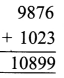Question 4.
You are given a magic square. Use the numbers form 1 to 9 only once and complete it so that every row and every column add up to 15.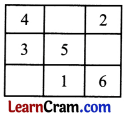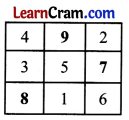Question 5.
A student was asked to write numeral for seventy six thousand and five. He wrote 7,605. Find the difference between the correct answer and his answer.
Correct answer = 7 6 0 0 5
Student answer = 7 6 0 5
Difference = 6 8 4 0 0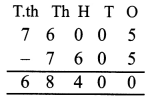Question 6.
The difference between two numbers is 98,506 and the greater number is 1,00,009. Find the smaller number.
Let the smaller number = x
greater number – smaller no. = Difference
1,00,009 – x = 48,506
-x = 48,506 – 1,00,009
x = 1,00,009 – 48, 506
Smaller number = 51,503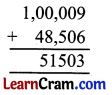Question 7.
Fill in the blanks.
(a) 4,128 + ___________ = 4,128
0

(b) ___________ + 82 + 71 = ___________ + 96 + 82
96; 71

(c) If 75 + 169 + 1,001 = 1,245 then 1,00 + 75 + 169 = ___________.
1,245

(d) 11,111 + 1,111 + 111 + 11 + 1 = ___________
12, 345

(e) ___________ – 0 = 785
785

(f) 4,500 – 1,500 = ___________
3,000

(g) 18,000 – ___________ = 8,000
10,000

(h) 7,500 + 2,500 = ___________
10,000

Question 1.
Solve the following:
(a) 6,000 + 6 + 16 =
6,022

(b) 2,007 – 207 = 1,300 + ?
500

(c) 21,483 – ? = 16,483
5,000

(d) The difference between the 2760 and 5000 is ___________.
5,000 – 2,760 = 2,240

(e) 90,000 – 2,786 = ?
87,214

Question 2.
(i) 27,566 + 30,647
Arrange in column.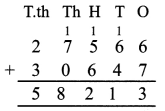(ii) 54,410 + 98,862
Arrange in column.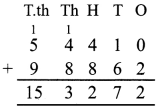(iii) 1,24,732 + 87,540
Arrange in column.Question 3.
Subtract the following:
(i) 53,400 and 37,423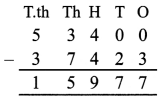(ii) 54,625 and 38,876(iii) Sixty six thousand and six from ninety thousand and twenty six.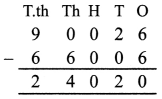(iv) 32,040 and 2,630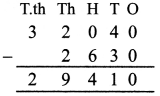Question 4.
What number should be subtracted from 32,055 to get 8,675?
Number should be difference of two number so. 32055 – 8675 = 23,380Question 5.
A number exceeds 2,48,545 by 17,605. What is that number?